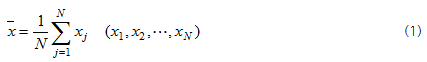Rescaling by Mean and Standard Deviation is used for rescaling through setting of arbitrary mean and standard deviation values, random variables of signals. Prior to description of this method, mean and standard deviation will be explained.

Mean and standard deviation are basic values determining the probable characteristics of signals. Mean value, also called as expected value, can be expressed as follow;Mean, calculated by dividing sum of all signal values by total number of signal data, indicates the middle value in the probability distribution graph. The probability distribution is the one expressed as sum of 1 showing how probability is distributed depending on random variables, when signals values are deemed as probability variables.

Prior to description of standard deviation, it is necessary to explain Variance.Variance is the value defining the degree of being distant from mean in the probability distribution graph. Standard deviation, the value calculated through root of variance, is defined as follow;Standard deviation is the value relating to form of the probability distribution graph. While referring to the following graph, meanings of individual values will be discussed again.

The middle value in the probability distribution graph is Mean, while the distribution degree of the probability distribution graph is Standard Deviation. In other words, lower standard deviation means narrower width of the probability distribution graph, indicating that data are centered on mean value. In short, lower value of standard deviation means that most data values are similar to the mean and the difference among values is not distinct. Probability distribution graph depending on changes of mean and standard deviation can be expressed as above.

Select data you want to apply this method in Analysis Window and click the icon of Rescaling by Mean and Standard Deviation in Analysis Tool Bar. This will display a dialogue box for setting of mean and standard deviation as shown in the following figure. Input values as you need and click Apply button. The graph is shown in Display Window and registered at Analysis Window.